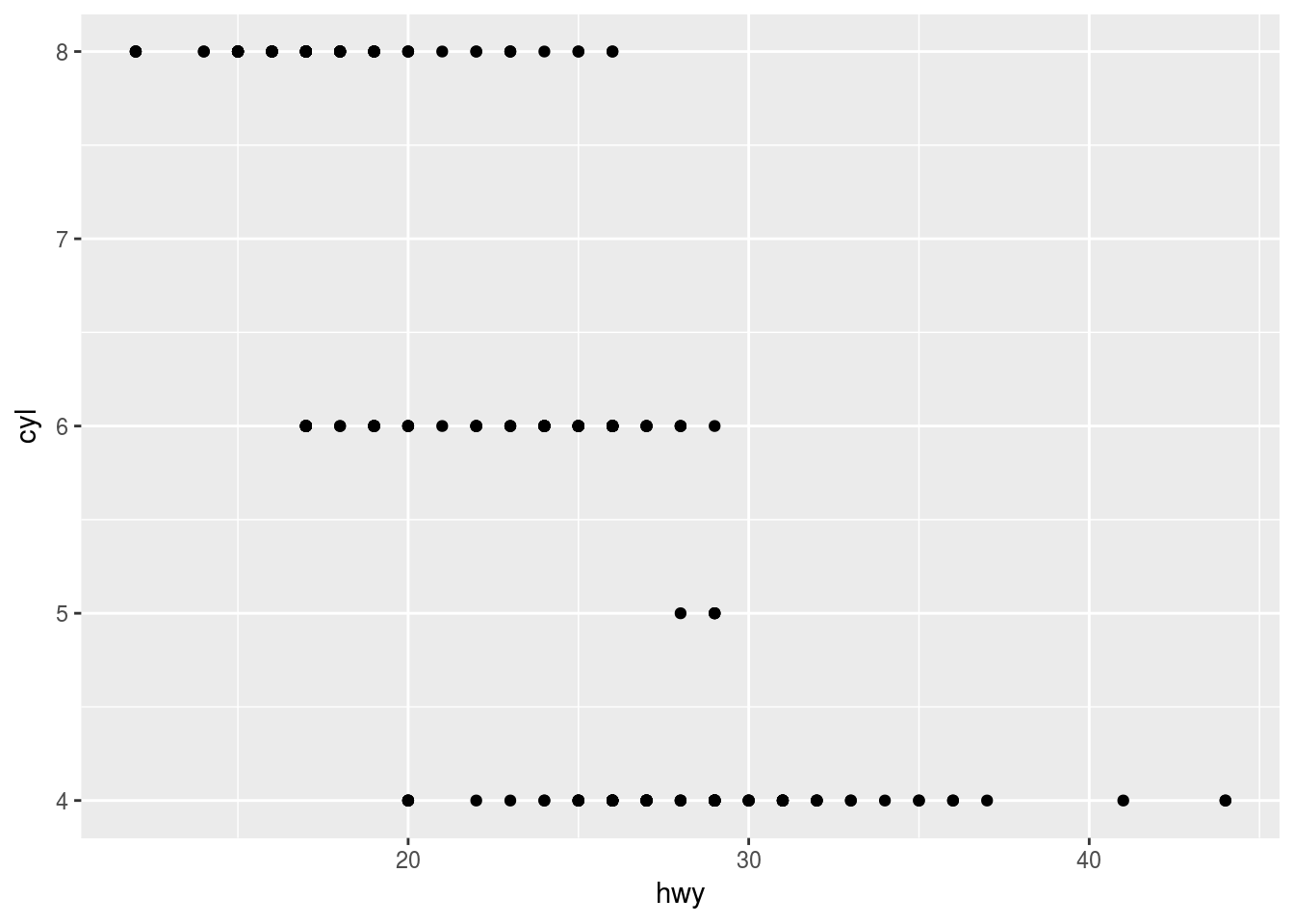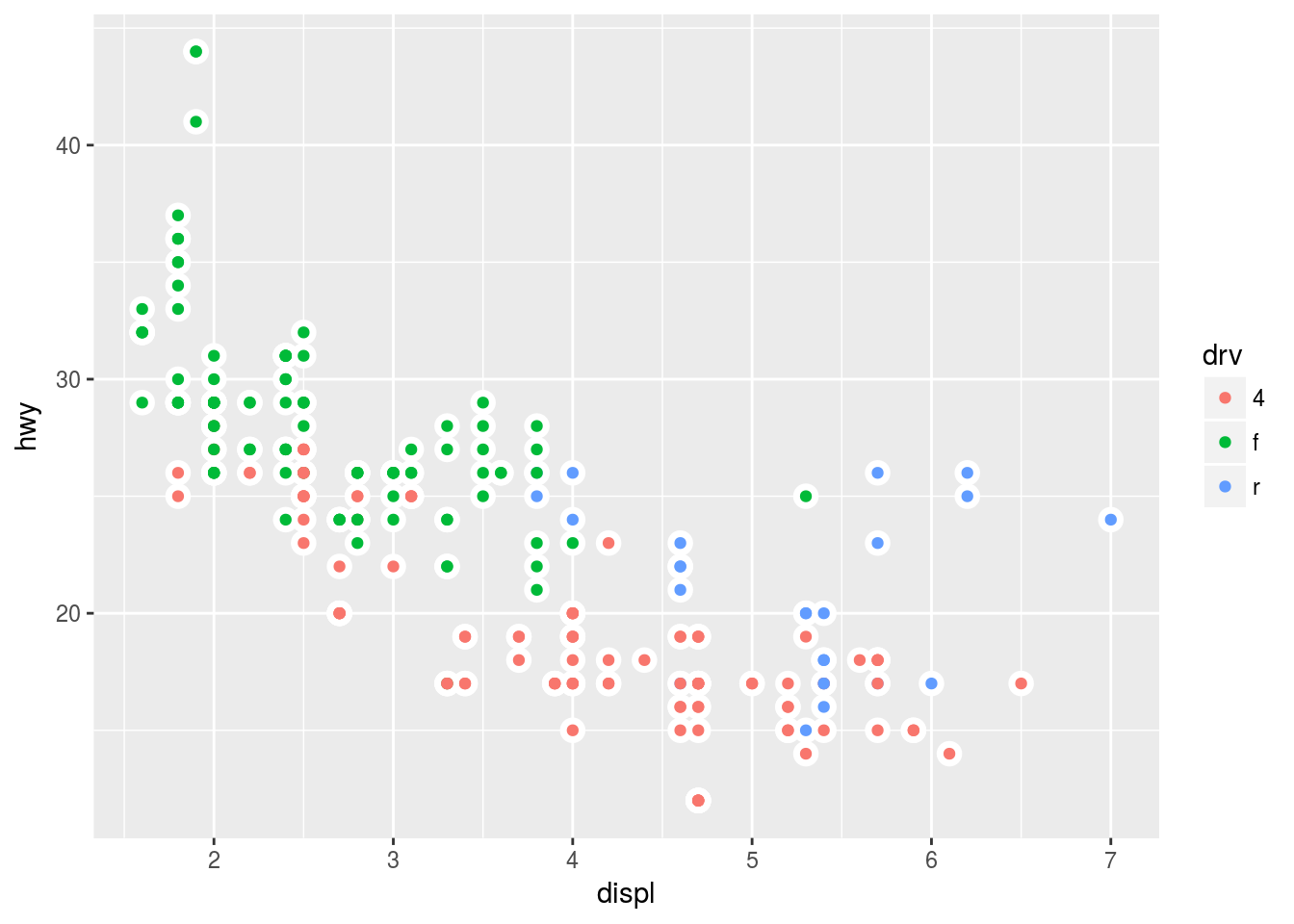# Chapter 3 Data visualisation

Author: Ron

``````library(tidyverse)
library(nycflights13)``````

## 3.1 3.2 First steps

### 3.2.4 Exercises

1. Make a scatter plot of hwy vs cyl.
``````ggplot(data = mpg, mapping = aes(x= hwy, y=cyl))+
geom_point()``````## 3.6 Geometric objects

### 3.6.1 Exercises

1. Recreate the R code necessary to generate the following graphs.
``````ggplot(data = mpg, aes(x = displ, y = hwy)) +
geom_point(color = "white", size = 4) +
geom_point(aes(color = drv))``````